天津大学 985 双一流 211

TianjinUniversity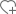关注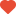已关注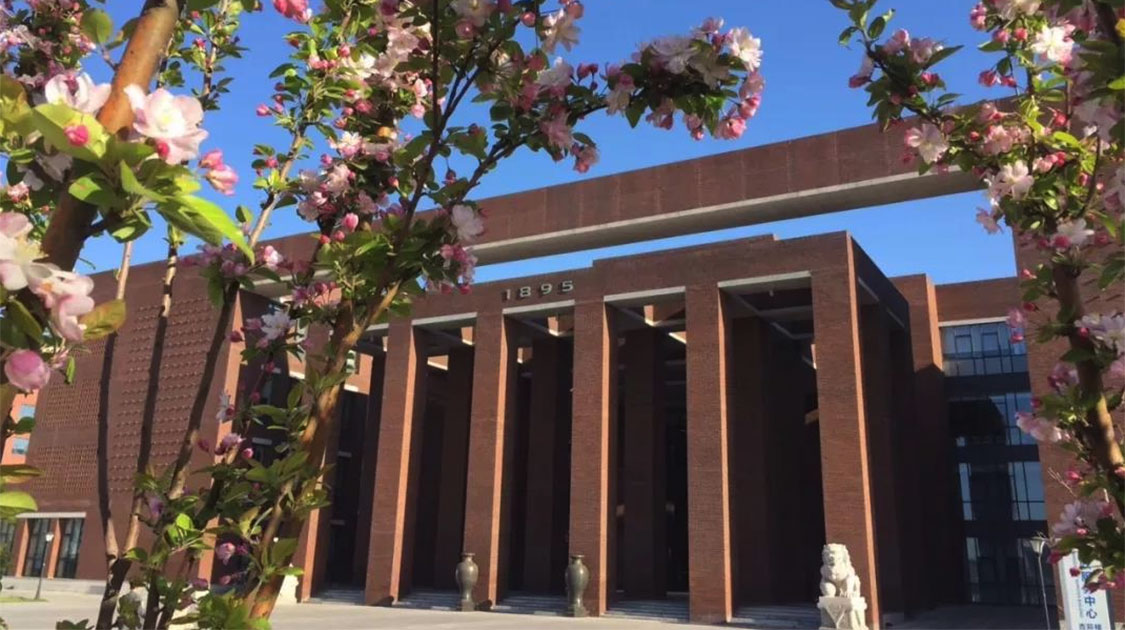•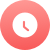1895

•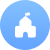公立

•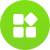理工

•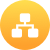教育部

•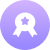本科

•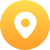天津-南开区

•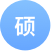178个

•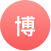126个

院校分数线

多方向招生特色专业

• 电子科学与技术
• 港口航道与海岸工程
• 工商管理
• 电子信息工程
• 化学工程与工艺
• 建筑学
• 测控技术与仪器
• 工程管理
• 电气工程及其自动化
• 数学与应用数学
• 材料科学与工程
• 机械设计制造及其自动化
• 土木工程
• 制药工程
• 船舶与海洋工程
• 应用化学
• 信息工程
• 水利水电工程
• 工程力学
• 材料成型及控制工程
• 热能与动力工程
• 通信工程
• 软件工程
• 建筑环境与设备工程
• 生物医学工程
• 环境工程院系/专业

• 机械设计制造及其自动化（本）
• 工程力学（本）
• 能源与动力工程（本）
• 工业设计（本）

• 测控技术与仪器（本）
• 光电信息科学与工程（本）
• 光电信息科学与工程(天南大合办)（本）
• 电子科学与技术(光电子技术方向)（本）
• 生物医学工程（本）

• 电气工程及其自动化（本）
• 自动化（本）
• 电子信息工程（本）
• 物联网工程（本）
• 通信工程（本）

• 电子科学与技术(固体电子与微电子)（本）
• 集成电路设计与集成系统（本）

• 土木工程（本）
• 水利水电工程（本）
• 港口航道及海岸工程（本）
• 船舶与海洋工程（本）

• 建筑学（本）
• 城乡规划（本）
• 环境设计（本）

• 化学工程与工艺（本）
• 制药工程（本）
• 食品科学与工程（本）
• 应用化学（本）
• 分子科学与工程（本）
• 过程装备与控制工程（本）
• 生物工程（本）

• 材料科学与工程（本）
• 功能材料（本）
• 材料成型及控制工程（本）

• 财务管理（本）
• 电子商务（本）
• 工程管理（本）
• 工商管理（本）
• 工业工程（本）
• 信息管理与信息系统（本）
• 信息管理与信息系统(保密管理方向)（本）
• 物流工程（本）
• 金融学（本）

• 应用物理学（本）
• 应用化学（本）

• 英语（本）
• 汉语言文学（本）

• 药学（本）

• 教育学（本）

• 建筑环境与能源应用工程（本）
• 环境工程（本）
• 环境科学（本）

• 计算机科学与技术（本）

• 软件工程（本）
• 动画（本）

• 生物科学（本）

• 海洋技术（本）

• 数学与应用数学（本）

• 法学（本）

大学排名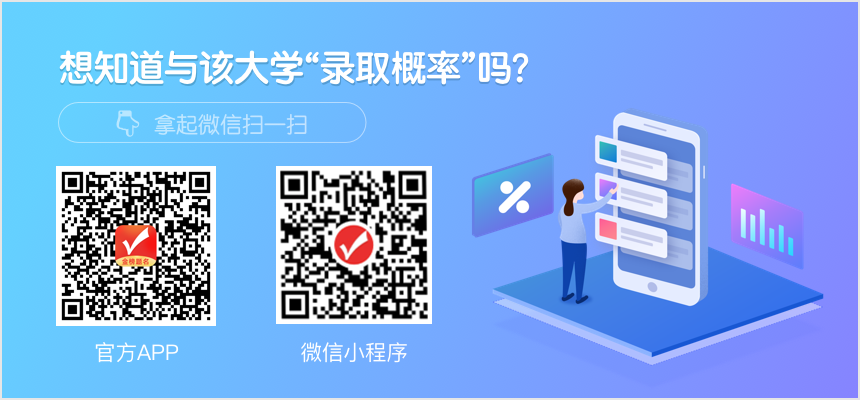知名校友

•李彦宏

•俞洪敏

•撒贝宁

•马云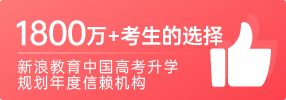400-181-5008

(免长话费 工作日 09:00-17:30)